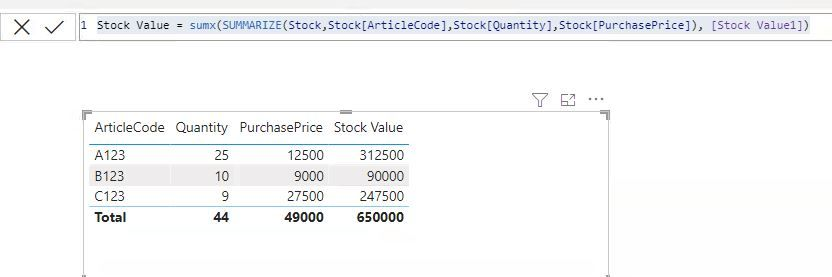cancel
Showing results for
Did you mean:New Member

## the sum of lines containing a multiplication is incorrect.

Hello,

I get, I don't think so complicated problem, just not solved.

Goal: I want to calculate the total stockvalue of a warehouse.

I have the following data available:

table: article

field: articlecode

table: stock

field: quantity

table: purchase

field: purchaesprice

By multiplying quantity x purchaseprice I get the stock value per item (articlecode). I get the total stock value by adding up all the lines per item.

The result I now get in the PowerBi report is as follows:

 Articlecode Quantity Purchaseprice Stockvalue A123 25 125,00 3.125,00 B123 10 90,00 900,00 C123 9 275,00 2.475,00 Total 44 490,00 21.560,00 Correct 6.500,00

So it's totally wrong. The Total is also the multiplication of the totals of quantity and purchase price. It should be as mentioned by Correct.

I think it has to do with Sum and Sumx, but I'm not sure what to do.

` `

1 ACCEPTED SOLUTIONHelper I

Hi

Create 2 measures as below.

1. Stock Value1 = sum(Stock[Quantity])*sum(Stock[PurchasePrice])

2. Stock Value = sumx(SUMMARIZE(Stock,Stock[ArticleCode],Stock[Quantity],Stock[PurchasePrice]), [Stock Value1])Please confirm if the solution works..!!

2 REPLIES 2Super User

Try this:

``````Stock Value =
VAR _A =
stock,
"Stockvalue", SUM( stock[quantity] ) * SUM( purchase[purchaesprice] )
)
RETURN
SUMX( _A, [Stockvalue] )``````

If this post helps, please consider accepting it as the solution to help the other members find it more quickly.Helper I

Hi

Create 2 measures as below.

1. Stock Value1 = sum(Stock[Quantity])*sum(Stock[PurchasePrice])

2. Stock Value = sumx(SUMMARIZE(Stock,Stock[ArticleCode],Stock[Quantity],Stock[PurchasePrice]), [Stock Value1])Please confirm if the solution works..!!